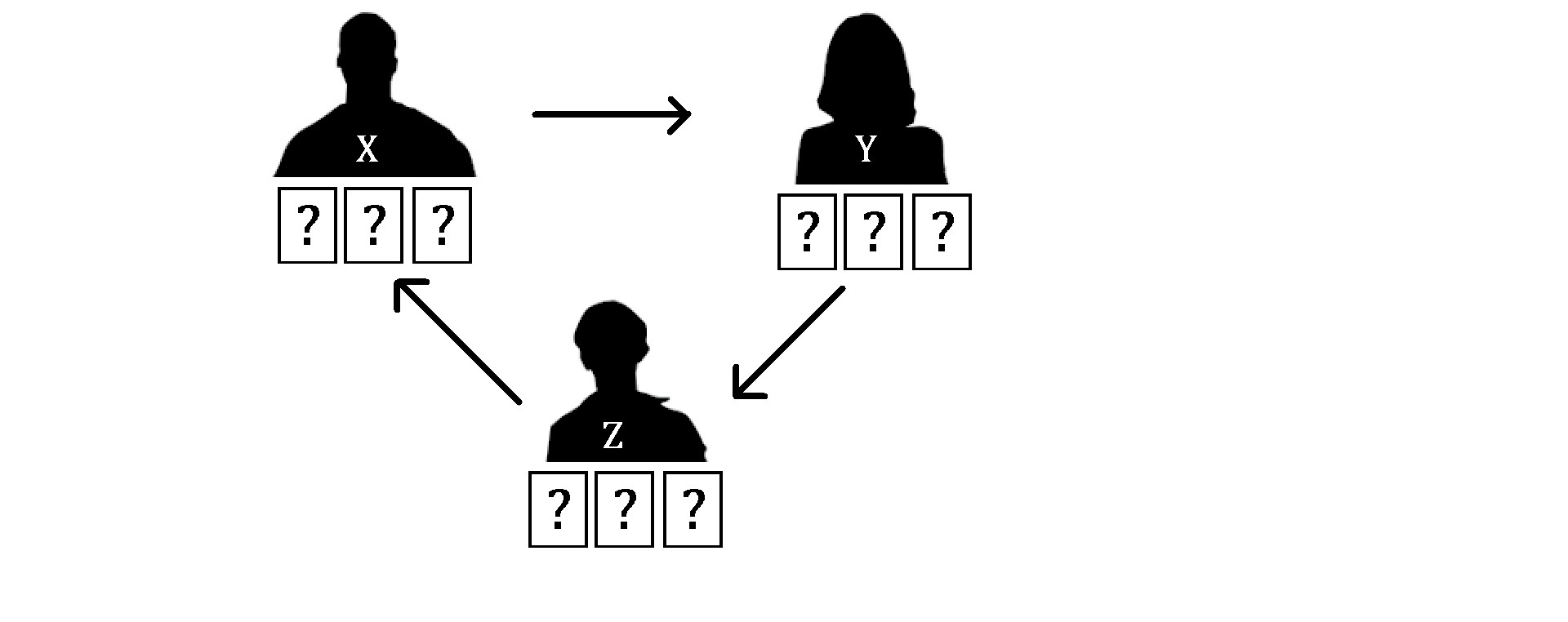# Equal Sum Game

Logic Level 4Mr. X, Mrs. Y, & Mr. Z were $3$ card players, each holding $3$ cards in their hands. All of them knew that the number on the card varied from $1$ to $9$ distinctly, but what they didn't realize was that the sums of numbers they had were all equal among the three of them.

To play this game, each of them would show one of their cards on the table, and whoever had the highest number would win the round. (Assuming $1$ was the lowest with no aces.) Taking turns as shown above, after each round ended, the beginner would shift to the next person. (For example, if the first round was X to Y to Z, then the next round would be Y to Z to X and so on.)

The $3$ players would play the $3$ rounds logically: They would use their highest "trump" card whenever they were absolutely sure they would win. On the other hand, they would opt their "medium" or lower card when they were unsure, and after seeing the opponent's high card, they would discard their lowest card instantly.

With Mr. X starting the game, the resulted winners were Mrs. Y in round #1, Mr. X in round #2, and Mr. Z in round #3 respectively. Interestingly, the sums of the revealed cards in each round were all equal to the original sum held in their hands!

If the alphabets $A$ - $I$ represented the values of the first to the last cards played respectively, what would be the value of the 9-digit $\overline{ABCDEFGHI}$?

×

Problem Loading...

Note Loading...

Set Loading...